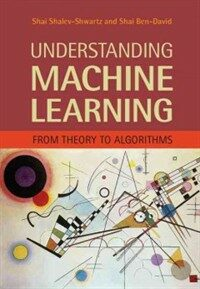> 상세정보

# 상세정보## Understanding machine learning : from theory to algorithms (1회 대출)

자료유형
단행본
개인저자
Shalev-Shwartz, Shai. Ben-David, Shai.
서명 / 저자사항
Understanding machine learning : from theory to algorithms / Shai Shalev-Shwartz, The Hebrew University, Jerusalem, Shai Ben-David, University of Waterloo, Canada.
발행사항
New York, NY, USA :   Cambridge University Press,   c2014.
형태사항
xvi, 397 p. : ill. ; 26 cm.
ISBN
9781107057135 (hardback) 1107057132 (hardback)
요약
"Machine learning is one of the fastest growing areas of computer science, with far-reaching applications. The aim of this textbook is to introduce machine learning, and the algorithmic paradigms it offers, in a principled way. The book provides an extensive theoretical account of the fundamental ideas underlying machine learning and the mathematical derivations that transform these principles into practical algorithms. Following a presentation of the basics of the field, the book covers a wide array of central topics that have not been addressed by previous textbooks. These include a discussion of the computational complexity of learning and the concepts of convexity and stability; important algorithmic paradigms including stochastic gradient descent, neural networks, and structured output learning; and emerging theoretical concepts such as the PAC-Bayes approach and compression-based bounds. Designed for an advanced undergraduate or beginning graduate course, the text makes the fundamentals and algorithms of machine learning accessible to students and non-expert readers in statistics, computer science, mathematics, and engineering"--
내용주기
Machine generated contents note: 1. Introduction; Part I. Foundations: 2. A gentle start; 3. A formal learning model; 4. Learning via uniform convergence; 5. The bias-complexity tradeoff; 6. The VC-dimension; 7. Non-uniform learnability; 8. The runtime of learning; Part II. From Theory to Algorithms: 9. Linear predictors; 10. Boosting; 11. Model selection and validation; 12. Convex learning problems; 13. Regularization and stability; 14. Stochastic gradient descent; 15. Support vector machines; 16. Kernel methods; 17. Multiclass, ranking, and complex prediction problems; 18. Decision trees; 19. Nearest neighbor; 20. Neural networks; Part III. Additional Learning Models: 21. Online learning; 22. Clustering; 23. Dimensionality reduction; 24. Generative models; 25. Feature selection and generation; Part IV. Advanced Theory: 26. Rademacher complexities; 27. Covering numbers; 28. Proof of the fundamental theorem of learning theory; 29. Multiclass learnability; 30. Compression bounds; 31. PAC-Bayes; Appendix A. Technical lemmas; Appendix B. Measure concentration; Appendix C. Linear algebra.
서지주기
Includes bibliographical references (p. 385-393) and index.
일반주제명
Machine learning. Algorithms.

### 소장정보

No. 소장처 청구기호 등록번호 도서상태 반납예정일 예약 서비스
No. 1 소장처 청구기호 006.31 S528u 등록번호 121246195 도서상태 대출가능 반납예정일 예약 서비스

### 컨텐츠정보

#### 목차

`Introduction -- I. Foundations -- A gentle start -- A formal learning model -- Learning via uniform convergence -- The bias-complexity tradeoff -- The VC-dimension -- Nonuniform learnability -- The runtime of learning -- II. From Theory to Algorithms -- Linear predictors -- Boosting -- Model selection and validation -- Convex learning problems -- Regularization and stability -- Stochastic gradient descent -- Support vector machines -- Kernel methods -- Multiclass, ranking, and complex prediction problems -- Decision trees -- Nearest neighbor -- Neural networks -- III. Additional Learning Models -- Online learning -- Clustering -- Dimensionality reduction -- Generative models -- Feature selection and generation -- IV. Advanced Theory -- Rademacher complexities -- Covering numbers -- Proof of the fundamental theorem of learning theory -- Multiclass learnability -- Compression bounds -- PAC-Bayes.`

### 관련분야 신착자료

#### 웹 브라우저 속 머신러닝 TensorFlow.js : 실전 예제로 배우는 텐서플로 머신러닝 모델

Sasaki, Kai (2021)

#### Quantum computing : how it works and how it could change the world

Katwala, Amit (2021)

#### Artificial neural networks / 3rd ed

Cartwright, Hugh M. (2021)

박유성 (2021)

정현성 (2020)

#### 빅데이터 개론 : analyse data / 4판(최신개정판)

한국소프트웨어기술인협회. 빅데이터전략연구소 (2021)

#### (Do it!) 클론 코딩 트위터 : 노마드 코더 니꼴라스와 만드는 트위터 서비스 : 코딩은 진짜를 만들어 보는 것!

Arévalo, Nicolás Serrano (2021)

이기훈 (2020)

허팝 (2020)

권미용 (2020)

#### 인공지능은 무엇이 되려 하는가 : AI의 가능성과 위험을 바라보는 석학 25인의 시선

Brockman, John (2021)

레이나 (2021)

민지영 (2021)

자토 (2020)

김진하 (2021)

이고잉 (2021)

박현경 (2020)

박혜린 (2020)